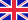•# Richard Feynman

Autor: Source: Wikipedia

Source: Wikipedia. Pages: 32. Chapters: Brownian ratchet, Cargo cult science, Feynman checkerboard, Feynman diagram, Feynman Long Division Puzzles, Feynman point, Feynman sprinkler, Foresight Institute Feynman Prize in Nanotechnology, Hellmann-Feynman theorem,... Viac o knihe

Na objednávku, dodanie 2-4 týždne

14.37 €

bežná cena: 16.90 €

## O knihe

Source: Wikipedia. Pages: 32. Chapters: Brownian ratchet, Cargo cult science, Feynman checkerboard, Feynman diagram, Feynman Long Division Puzzles, Feynman point, Feynman sprinkler, Foresight Institute Feynman Prize in Nanotechnology, Hellmann-Feynman theorem, Infinity (film), Joan Feynman, One-electron universe, Ralph Leighton, Tuva or Bust!, Wheeler-Feynman absorber theory. Excerpt: In theoretical physics, Feynman diagrams are pictorial representations of the mathematical expressions governing the behavior of subatomic particles. The scheme is named for its inventor, Nobel Prize-winning American physicist Richard Feynman, and was first introduced in 1948. The interaction of sub-atomic particles can be complex and difficult to understand intuitively, and the Feynman diagrams allow for a simple visualization of what would otherwise be a rather arcane and abstract formula. As David Kaiser writes, "since the middle of the 20th century, theoretical physicists have increasingly turned to this tool to help them undertake critical calculations," and as such "Feynman diagrams have revolutionized nearly every aspect of theoretical physics". While the diagrams are applied primarily to quantum field theory, they can also be used in other fields, such as solid-state theory. Feynman proposed an interpretation of the positron as if it were an electron moving backward in time. Thus Feynman diagrams contain both a space axis and a time axis, and antiparticles are interpreted as moving forward in space but backward in time. The calculation of probability amplitudes in theoretical particle physics requires the use of rather large and complicated integrals over a large number of variables. These integrals do, however, have a regular structure, and may be represented graphically as Feynman diagrams. A Feynman diagram is a contribution of a particular class of particle paths, which join and split as described by the diagram. More precisely, and technically, a Feynman diagram is a graphical representation of a perturbative contribution to the transition amplitude or correlation function of a quantum mechanical or statistical field theory. Within the canonical formulation of quantum field theory, a Feynman diagram represents a term in the Wick's expansion of the perturbative S-matrix. Alternatively, the path integral formulation of quantum field theory represents the

• Vydavateľstvo: Books LLC, Reference Series
• Rok vydania: 2013
• Formát: Paperback
• Rozmer: 246 x 189 mm
• Jazyk: Anglický jazyk
• ISBN: 9781156692059

Generuje redakčný systém BUXUS CMS spoločnosti ui42.